• 主要为大家详细介绍了C语言版二值图像统计连通区域的相关资料，具有一定的参考价值，感兴趣的小伙伴们可以参考一下
• 国外一个大佬的论文翻译过来的版本 关于图像小波边缘统计模型 小波联合统计模型法 图像的压缩 恢复 合成
• 文章目录数学期望方差协方差（矩阵）与相关系数矩图像矩Raw MomentsCentral Moments参考资料 我们可以将一幅数字图像视为一个 二维函数I(x,y)I(x,y)I(x,y) ，其中x和y是空间坐标，在x-y平面中的任意空间坐标 (x,y)(x...


文章目录
数学期望方差协方差（矩阵）与相关系数矩图像矩Raw MomentsCentral Moments

参考资料

我们可以将一幅数字图像视为一个
二维函数

I

(

x

,

y

)

I(x,y)

，其中x和y是空间坐标，在x-y平面中的任意空间坐标

(

x

,

y

)

(x,y)

上的
幅值

I

x

y

I_{xy}

称为该点
图像的灰度、亮度或强度。

I

x

,

y

=

I

(

x

,

y

)

I_{x,y} = I(x,y)

下面以

I

x

y

I_{xy}

作为随机变量，分析二维数字图像的 统计特征。
数学期望
数学期望（Expectation） 就是随机变量 以概率为权数的加权平均值，以 级数 的形式表示为

E

(

X

)

=

∑

k

=

1

∞

x

k

p

k

E(X) = \sum_{k=1}^{\infty} x_k p_k

一幅数字图像的数学期望为其 灰度平均值，即所有像元灰度值的算数平均值

I

ˉ

=

1

M

⋅

N

∑

x

=

1

M

∑

y

=

1

N

I

(

x

,

y

)

\bar{I} = \frac{1}{M \cdot N} \sum_{x=1}^{M} \sum_{y=1}^{N} I(x,y)

方差
方差（variance） 是对随机变量离散程度的度量。

D

(

x

)

=

E

[

X

−

E

(

X

)

]

2

=

E

(

X

2

)

−

[

E

(

X

)

]

2

D(x) = E[X-E(X)]^2 = E(X^2) - [E(X)]^2

称

D

(

X

)

\sqrt{D(X)}

为随机变量的 标准差（standard deviation），或 均方差（mean square deviation），记为

σ

(

X

)

\sigma(X)

二维数字图像的 灰度方差 反应的是图像中各个像素的灰度值与整个图像平均灰度值的离散程度，与图像的 对比度 有关。如果图片对比度小，那方差就小；如果图片对比度很大，那方差就大。

v

a

r

(

I

)

=

1

M

⋅

N

∑

x

=

1

M

∑

y

=

1

N

[

I

(

x

,

y

)

−

I

ˉ

]

var(I) = \frac{1}{M \cdot N} \sum_{x=1}^{M} \sum_{y=1}^{N} \left[ I(x,y) - \bar{I} \right]

协方差（矩阵）与相关系数
随机变量X、Y的 协方差（covariance） 为

c

o

v

(

X

,

Y

)

=

E

{

[

X

−

E

(

X

)

]

[

Y

−

E

(

Y

)

]

}

=

E

[

X

Y

]

−

E

(

X

)

E

(

Y

)

=

∑

i

∑

j

[

x

i

−

E

(

X

)

]

[

y

j

−

E

(

Y

)

]

p

i

j

\begin{aligned} cov(X,Y) &amp;= E \{ [X-E(X)][Y-E(Y)] \} = E[XY] - E(X)E(Y) \\ &amp;= \sum_{i} \sum_{j} [x_i-E(X)] [y_j-E(Y)] p_{ij} \end{aligned}

协方差矩阵 可表示为

Σ

=

[

σ

11

σ

12

…

σ

1

n

σ

21

σ

22

…

σ

2

n

⋮

⋮

⋱

⋮

σ

n

1

σ

n

2

…

σ

n

n

]

\Sigma = \begin{bmatrix} \sigma_{11} &amp; \sigma_{12} &amp; \ldots &amp; \sigma_{1n} \\ \sigma_{21} &amp; \sigma_{22} &amp; \ldots &amp; \sigma_{2n} \\ \vdots &amp; \vdots &amp; \ddots &amp; \vdots \\ \sigma_{n1} &amp; \sigma_{n2} &amp; \ldots &amp; \sigma_{nn} \\ \end{bmatrix}

其中，

σ

i

j

=

c

o

v

(

X

i

,

X

j

)

\sigma_{ij} = cov(X_i, X_j)

随机变量X、Y的 相关系数（correlation coefficient） 或 标准协方差（standard covariance） 为

ρ

X

Y

=

c

o

v

(

X

,

Y

)

D

(

X

)

D

(

Y

)

\rho_{XY} = \frac{ cov(X,Y) }{ \sqrt{D(X)} \sqrt{D(Y)} }

数字图像

I

A

I_A

和

I

B

I_B

的协方差为

c

o

v

(

I

A

,

I

B

)

=

1

M

⋅

N

∑

x

=

1

M

∑

y

=

1

N

[

I

A

(

x

,

y

)

−

I

A

ˉ

]

[

I

B

(

x

,

y

)

−

I

B

ˉ

]

cov(I_A, I_B) = \frac{1}{M \cdot N} \sum_{x=1}^{M} \sum_{y=1}^{N} [ I_A(x,y) - \bar{I_A} ] [ I_B(x,y) - \bar{I_B} ]

图像的相关系数表征的是两个不同波段图像的所含信息的重叠程度，相关系数越大，重叠度越高，反之越低。

ρ

I

A

I

B

=

∑

x

=

1

M

∑

y

=

1

N

[

I

x

y

A

−

I

A

ˉ

]

[

I

x

y

B

−

I

B

ˉ

]

(

∑

x

=

1

M

∑

y

=

1

N

[

I

x

y

A

−

I

A

ˉ

]

)

1

/

2

(

∑

x

=

1

M

∑

y

=

1

N

[

I

x

y

B

−

I

A

ˉ

]

)

1

/

2

\rho_{I_A I_B} = \frac {\sum_{x=1}^{M} \sum_{y=1}^{N} [ I^A_{xy} - \bar{I_A} ] [ I^B_{xy} - \bar{I_B} ]} { \bigg(\sum_{x=1}^{M} \sum_{y=1}^{N} [ I^A_{xy} - \bar{I_A} ]\bigg)^{1/2} \bigg(\sum_{x=1}^{M} \sum_{y=1}^{N} [ I^B_{xy} - \bar{I_A} ]\bigg)^{1/2} }

下面计算 lena图像 灰度图 和 高斯模糊图 的相关系数img = Image.open('lena.bmp').convert('L')
im = np.asarray(img)
im_blur = ndimage.gaussian_filter(im, 4)
a = np.corrcoef(im.flatten(), im_blur.flatten())
print a

计算得到其相关系数为 0.95346
矩

X

X

的

k

k

阶原点矩，简称

k

k

阶矩：

E

[

X

k

]

E[X^k]

X

X

的

k

k

阶中心矩：

E

[

X

−

E

(

X

)

]

k

E[X-E(X)]^k

X

X

和

Y

Y

的

k

+

l

k+l

阶混合矩：

E

[

X

k

Y

l

]

E [ X^k Y^l ]

X

X

和

Y

Y

的

k

+

l

k+l

阶混合中心矩：

E

{

[

X

−

E

(

X

)

]

k

[

Y

−

E

(

Y

)

]

l

}

E \{ [X-E(X)]^k [Y-E(Y)]^l \}

显然，

X

X

的数学期望

E

(

X

)

E(X)

是

X

X

的一阶原点矩，方差

D

(

X

)

D(X)

是

X

X

的二阶中心矩，协方差

c

o

v

(

X

,

Y

)

cov(X,Y)

是

X

X

和

Y

Y

的 1+1阶混合中心矩
图像矩
图像的矩（Image Moments）主要表征了图像区域的几何特征，又称为 几何矩。
Raw Moments
二维灰度图像

I

I

的矩定义为

M

p

q

=

∑

x

∑

y

x

p

y

q

I

(

x

,

y

)

,

p

,

q

∈

{

0

,

1

,

2

,

…

}

M_{pq} = \sum_{x} \sum_{y} x^p y^q I(x,y), \quad p,q \in \{ 0,1,2, \ldots \}

零阶矩 为

M

00

=

∑

x

∑

y

I

(

x

,

y

)

M_{00} = \sum_{x} \sum_{y} I(x,y)

当图像为二值图时，

M

00

M_{00}

就是这个图像上白色区域的总和；因此，

M

00

M_{00}

可以用来求二值图像（轮廓、连通域）的面积
一阶矩 为

M

10

=

∑

x

∑

y

x

⋅

I

(

x

,

y

)

M_{10} = \sum_{x} \sum_{y} x \cdot I(x,y)

M

01

=

∑

x

∑

y

y

⋅

I

(

x

,

y

)

M_{01} = \sum_{x} \sum_{y} y \cdot I(x,y)

当图像为二值图时，

I

I

只有 0 和 1 两个值，

M

10

M_{10}

就是图像上所有白色区域 x坐标值 的累加一阶矩可以用来求图像的 质心（Centroid），这种方法对噪声不太敏感:

(

x

c

,

y

c

)

=

(

M

10

M

00

,

M

01

M

00

)

(x_c, y_c) = \bigg( \frac{M_{10}}{M_{00}}, \frac{M_{01}}{M_{00}} \bigg)

一阶矩还可以用来求 图像块几何中心的方向，即几何中心

O

O

与 质心

C

C

连接的 方向向量

O

C

⃗

\vec{OC}

的方向（计算中

x

,

y

x,y

均以几何中心

O

O

为原点）:

θ

=

a

r

c

t

a

n

(

M

01

M

10

)

\theta = arctan( \frac{M_{01}}{M_{10}} )

通过 取以关键点kp为几何中心的图像块 计算其 一阶矩 进而计算 该点方向，示例代码如下：
int m01 = 0;
int m10 = 0;
for(int y=-half_patch_size; y<half_patch_size; ++y){
for(int x=-half_patch_size; x<half_patch_size; ++x){
m01 += y * image.at<uchar>(kp.pt.y+y, kp.pt.x+x);
m10 += x * image.at<uchar>(kp.pt.y+y, kp.pt.x+x);
}
}
kp.angle = std::atan2(m01, m10)/CV_PI*180.0;

二阶矩 为

M

20

=

∑

x

∑

y

x

2

⋅

I

(

x

,

y

)

M_{20} = \sum_{x} \sum_{y} x^2 \cdot I(x,y)

M

02

=

∑

x

∑

y

y

2

⋅

I

(

x

,

y

)

M_{02} = \sum_{x} \sum_{y} y^2 \cdot I(x,y)

M

11

=

∑

x

∑

y

x

⋅

y

⋅

I

(

x

,

y

)

M_{11} = \sum_{x} \sum_{y} x \cdot y \cdot I(x,y)

Central Moments

μ

p

q

=

∑

x

∑

y

(

x

−

x

c

)

p

(

y

−

y

c

)

q

I

(

x

,

y

)

,

p

,

q

∈

{

0

,

1

,

2

,

…

}

\mu_{pq} = \sum_{x} \sum_{y} (x-x_c)^p (y-y_c)^q I(x,y), \quad p,q \in \{ 0,1,2, \ldots \}

利用二阶中心矩可以求图像的方向，图像的 协方差矩阵 为

c

o

v

[

I

(

x

,

y

)

]

=

[

μ

20

′

μ

11

′

μ

11

′

μ

02

′

]

cov[I(x,y)] = \begin{bmatrix} \mu_{20}&#x27; &amp; \mu_{11}&#x27; \\ \mu_{11}&#x27; &amp; \mu_{02}&#x27; \end{bmatrix}

求得图像方向为

θ

=

1

2

a

r

c

t

a

n

(

2

μ

11

′

μ

20

′

−

μ

02

′

)

\theta = \frac{1}{2} arctan(\frac{2\mu_{11}&#x27;}{\mu_{20}&#x27;-\mu_{02}&#x27;})

其中

μ

20

′

=

μ

20

μ

00

=

M

20

M

00

−

x

c

2

\mu_{20}&#x27; = \frac{\mu_{20}}{\mu_{00}} = \frac{M_{20}}{M_{00}} - x_c^2

μ

02

′

=

μ

02

μ

00

=

M

02

M

00

−

y

c

2

\mu_{02}&#x27; = \frac{\mu_{02}}{\mu_{00}} = \frac{M_{02}}{M_{00}} - y_c^2

μ

11

′

=

μ

11

μ

00

=

M

11

M

00

−

x

c

y

c

\mu_{11}&#x27; = \frac{\mu_{11}}{\mu_{00}} = \frac{M_{11}}{M_{00}} - x_c y_c

参考资料
《概率论与数理统计》Image moment (wikipedia)ORB: an efficient alternative to SIFT or SURF
展开全文• 直方图的计算方法为遍历图像的全部像素并累计每...在这种情况下使用积分图像将极大地提高统计图像子区域像素的效率。积分图像在程序中应用非常广泛。本文为学习笔记，实现书中给出的利用积分图像计算自适应阈值的例子。

OpenCV—使用积分图像统计像素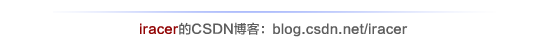本文为对《OpenCV计算机视觉编程攻略（第二版）》4.8节积分图像部分的学习笔记。

1.积分图像的基本概念
（1）为什么要用积分图像？
直方图的计算方法为遍历图像的全部像素并累计每个强度值在图像中出现的次数。有时仅需要计算图像中某个特定区域的直方图，而如果需要计算图像中多个区域的直方图，这些计算过程将变得非常耗时。在这种情况下使用积分图像将极大地提高统计图像子区域像素的效率。积分图像在程序中应用非常广泛。
（2）什么是积分图像？
积分图像的定义：取图像左上侧的全部像素计算累加和，并用这个累加和替换图像中的每一个像素，使用这种方式得到的图像称为积分图像。
（3）如何计算积分图像？
计算积分图像的函数：C++接口

void integral(InputArray image,OutputArray sum, int sdepth=-1 )
void integral(InputArray image,OutputArray sum, OutputArray sqsum, int sdepth=-1 )
void integral(InputArray image,OutputArray sum, OutputArray sqsum, OutputArray tilted,int sdepth=-1 )

函数参数：

image   输入W×H源图像，8bit字符型，或32bit、64bit浮点型矩阵
sum     输出(W+1)×(H +1)积分图像，32bit整型或32bit、64bit浮点型矩阵
sqsum   输出(W+1)×(H +1)平方积分图像，双精度浮点型矩阵。
tilted     输出旋转45°的(W+1)×(H +1)积分图像，数据类型同sum
sdepth  积分图像sum或titled的位深度：CV_32S、CV_32F或CV_64F

根据定义，计算源图像的积分图像的函数执行如下操作：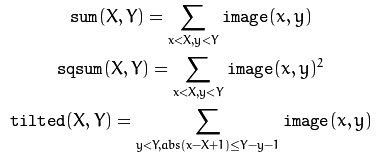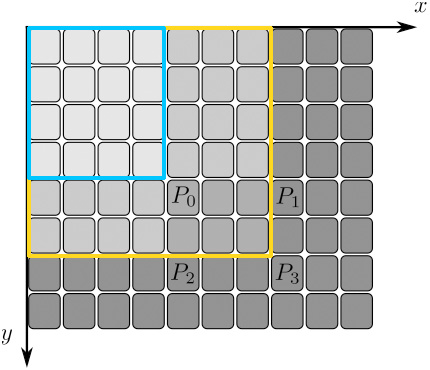按照上面的公式，P0点的积分值为蓝色方框中像素的强度累加和，P3点的积分像素为黄色方框内像素点的强度累加和。从上图可知，想要从原始图像获得积分图像，只需要对整幅图像扫描一次。这是因为对同一行（列）的相邻两像素，当前像素的积分值等于上一像素的积分值加上当前像素所在列（行）的左上侧累计值。可见，积分图像是一个包含像素累加和的新图像。为了防止累加和过大溢出，积分图像的值通常都使用int类型变量（CV_32S）或float类型变量（CV_32F）。
对于多通道图像，每个通道都将独立累积计算积分图像。
（4）如何使用积分图像？
假设一幅图像中4个点ABCD，其积分图像中A(x1,y1)点的值为其左上侧所有像素的值的累加和，也就是蓝色区域中所有像素点的值累加和。同理积分图像中的B(x2,y1)、C(x1,y2)、D(x2,y2)点值分别是绿色、紫色和黄色区域像素值的累加和。ABCD四点的位置关系在右下角图所示。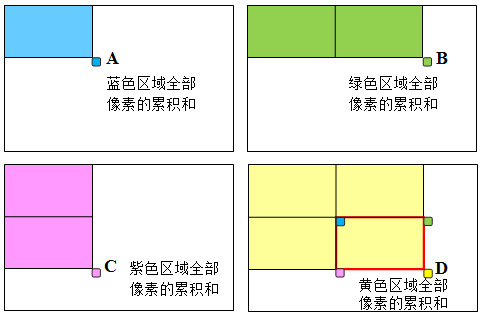那么如果需要计算由ABCD组成的ROI的累加值就只需要使用如下公式：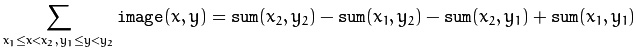即：D-C-B+A。显然，计算量不受区域尺寸的影响。所以，如果需要在多个尺寸的区域上计算像素累加和，最好采用积分图像。
2.利用积分图像进行自适应阈值分割
局部阈值，即根据每个像素的邻域计算阈值。这种策略叫做自适应阈值化，包括将每个像素的值与邻域的平均值进行比较。如果某像素的值与它的局部平均值差别很大，就会被当做异常值在阈值化过程中剔除。因此，自适应阈值化需要计算每个像素周围的局部平均值。这需要多次计算图像窗口的累计值，可以通过积分图像来提高计算效率。
首先，读取一幅图片

cv::Mat image= cv::imread("f:\\images\\book.jpg",0);
if (!image.data)
return 0;
// rotate the image for easier display
cv::transpose(image, image);
cv::flip(image, image, 0);

// display original image
cv::namedWindow("Original Image");
cv::imshow("Original Image",image);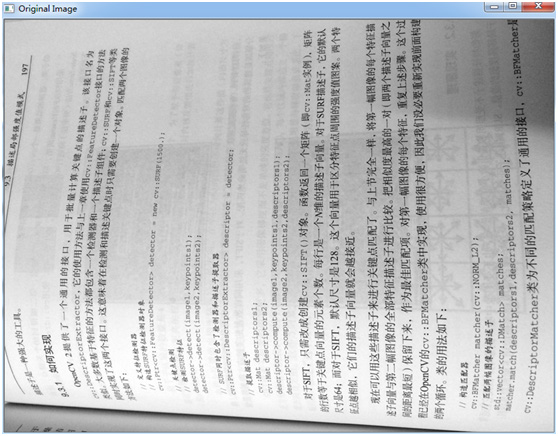原始图像
观察一下使用固定阈值分割得到的结果

// using a fixed threshold
cv::Mat binaryFixed;
cv::threshold(image,binaryFixed,180,255,cv::THRESH_BINARY);//固定阈值分割
cv::namedWindow("Fixed Threshold");
cv::imshow("Fixed Threshold",binaryFixed);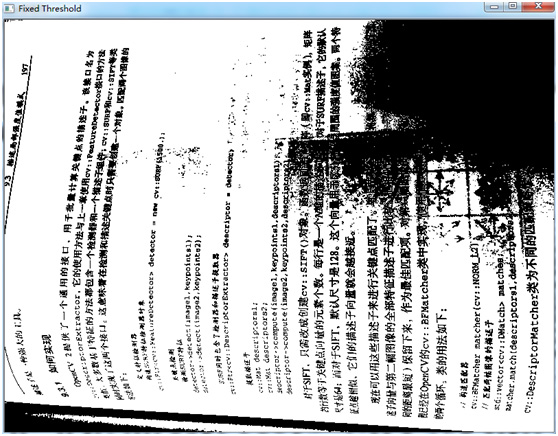使用固定阈值分割的结果
显然，固定阈值难以完成这个任务，难以给出不同光照区域的最佳分割。
对于这类问题，可以使用自适应阈值分割。实现自适应阈值分割有很多方法，最简单的就是直接使用opencv中的自适应阈值分割函数。

// using as adaptive threshold
int blockSize= 21; // size of the neighborhood
int threshold=10;  // pixel will be compared to (mean-threshold)

255,               // max value for output
cv::THRESH_BINARY, // threshold type
blockSize,         // size of the block
threshold);        // threshold used
cv::imshow("Adaptive Threshold",binaryAdaptive);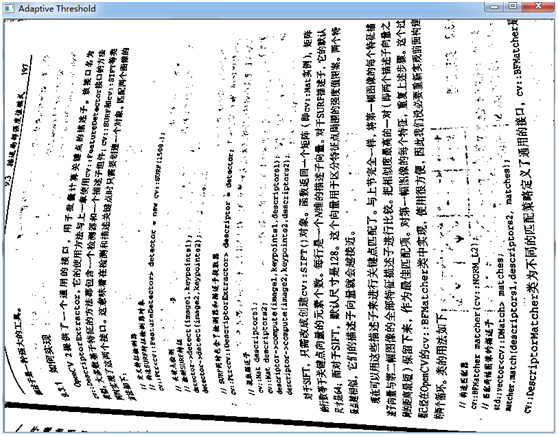（2）使用积分图像integral()实现自适应阈值分割
当然，也可以自己写程序实现自适应阈值分割

// compute integral image
IntegralImage<int,1> integral(image);//IntegralImage为计算积分图像的方法封装的模板类，实例化一个变量

cv::Mat binary= image.clone();//深度复制图像

// 使用指针遍历图像来计算积分图像
int nl= binary.rows; // number of lines
int nc= binary.cols; // total number of elements per line

// compute integral image
cv::Mat iimage;
cv::integral(image,iimage,CV_32S);//计算积分图像

// for each row
int halfSize= blockSize/2;
<span style="white-space:pre">	</span>for (int j=halfSize; j<nl-halfSize-1; j++) {

// get the address of row j
uchar* data= binary.ptr<uchar>(j);
int* idata1= iimage.ptr<int>(j-halfSize);
int* idata2= iimage.ptr<int>(j+halfSize+1);

// for pixel of a line
for (int i=halfSize; i<nc-halfSize-1; i++) {

// compute sum
int sum= (idata2[i+halfSize+1]-idata2[i-halfSize]-
idata1[i+halfSize+1]+idata1[i-halfSize])/(blockSize*blockSize);

if (data[i]<(sum-threshold))
data[i]= 0;
else
data[i]=255;
}
}

cv::imshow("Adaptive Threshold (integral)",binary);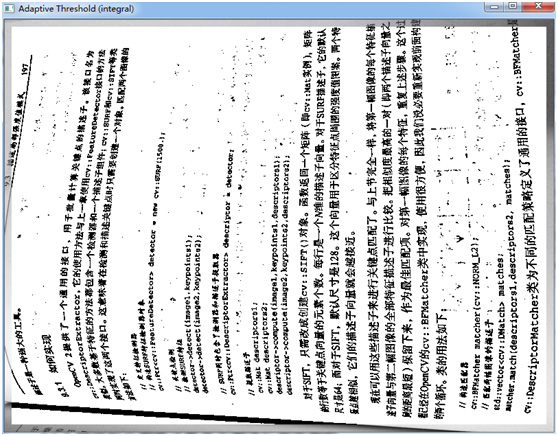在积分图像上实现自适应阈值分割

#if !defined IINTEGRAL
#define IINTEGRAL

#include <opencv2/core/core.hpp>
#include <opencv2/highgui/highgui.hpp>
#include <opencv2/imgproc/imgproc.hpp>

#include <vector>

template <typename T, int N> //将积分计算过程封装进模板类<T>为向量数据类型，<N>为向量数量
class IntegralImage {

cv::Mat integralImage;

public:

IntegralImage(cv::Mat image) {

// (costly) computation of the integral image
cv::integral(image,integralImage,cv::DataType<T>::type);
}

// compute sum over sub-regions of any size from 4 pixel access
cv::Vec<T,N> operator()(int xo, int yo, int width, int height) {

// window at (xo,yo) of size width by height
return (integralImage.at<cv::Vec<T,N> >(yo+height,xo+width)//Vec<T,N> ,T为类型，N为向量元素数量
-integralImage.at<cv::Vec<T,N> >(yo+height,xo)
-integralImage.at<cv::Vec<T,N> >(yo,xo+width)
+integralImage.at<cv::Vec<T,N> >(yo,xo));
}

// compute sum over sub-regions of any size from 4 pixel access
cv::Vec<T,N> operator()(int x, int y, int radius) {

// square window centered at (x,y) of size 2*radius+1
}
};

// convert to a multi-channel image made of binary planes
// nPlanes must be a power of 2
void convertToBinaryPlanes(const cv::Mat& input, cv::Mat& output, int nPlanes) {

// number of bits to mask out
int n= 8-static_cast<int>(log(static_cast<double>(nPlanes))/log(2.0));
// mask used to eliminate least significant bits

// create a vector of 16 binary images
std::vector<cv::Mat> planes;
// reduce to nBins bins by eliminating least significant bits

// compute each binary image plane
for (int i=0; i<nPlanes; i++) {

// 1 for each pixel equals to i<<shift
planes.push_back((reduced==(i<<n))&0x1);
}

// create multi-channel image
cv::merge(planes,output);
}

#endif

（3）使用图像运算符来实现自适应阈值分割
还可以使用OpenCV的图像运算符来实现自适应阈值分割的过程。

// adaptive threshold using image operators
cv::Mat filtered;
cv::Mat binaryFiltered;
cv::boxFilter(image,filtered,CV_8U,cv::Size(blockSize,blockSize));
filtered= filtered-threshold;
binaryFiltered= image>= filtered;
通过实验，上述3中方法中，使用第一种自适应阈值化函数花费的时间较少。

转载请注明：iracer的CSDN博客 http://blog.csdn.net/iracer/article/details/49029239

新书终于面市啦，《机器学习原理与编程实战》连接原理与实战：
https://blog.csdn.net/iracer/article/details/116051674?spm=1001.2014.3001.5501展开全文opencv C++
• ## GDAL计算栅格图像统计值相关说明

万次阅读 热门讨论 2013-01-11 23:15:00
一、 简介 在图像处理的过程中，会经常获取图像统计值来进行一些计算。这里说的图像统计值指：直方图，最大值，最小值，均值和方差这几个。下面分别对怎么使用GDAL来计算这些值做一个简单的说明，希望对大家...
一、        简介
在图像处理的过程中，会经常获取图像的统计值来进行一些计算。这里说的图像的统计值指：直方图，最大值，最小值，均值和方差这几个。下面分别对怎么使用GDAL来计算这些值做一个简单的说明，希望对大家有用。
下面将分为三个部分进行说明，分别是：直方图统计、极值统计、均值标准方差统计。
二、        统计直方图
关于直方图，这里不多介绍。这里主要对如何使用GDAL获取直方图进行说明。使用GDAL获取直方图的函数叫做GDALRasterBand::GetHistogram()，下面对这个函数的参数进行一个大致的说明。函数GDALRasterBand::GetHistogram的定义如下：

CPLErrGDALRasterBand::GetHistogram   (
double dfMin,
double dfMax,
int nBuckets,
int *     panHistogram,
int bIncludeOutOfRange,
int bApproxOK,
GDALProgressFunc   pfnProgress,
void *   pProgressData
)
为了更形象的说明直方图，将结合图1进行说明。图1是一个8bit的图像的全部直方图像素值的一个列表，每个横向的线段代表16个灰度值间隔。图中的数字表示右下角交叉处的灰度值（图中红色圈的位置表示的是0和256的位置，可能有点不清楚，瞪大眼睛仔细看～～）。图1 直方图示例

第一个参数和第二个参数，即dfMin和dfMax，这两个参数的意思是指统计直方图的最小值和最大值，在图1中的话，就是这个横向数轴的一个区间。比如一个8bit的图像，你想统计所有的直方图，那么这两个值可以设置为-0.5和255.5。至于为什么不是0和255，是因为GDAL的这个函数是个开区间，就是如果设置为0和255的话，那么0和255这两个值是不会被统计的，所以需要把最小值往小减小一点，最大值往大增加一点。-0.5和255.5只是我的习惯，你也可以用-0.1和255.1，只要把0和255包括进去即可。如果你只想统计128到255之间的直方图，那么把最小值设置为127.5即可；如果想统计0到128之间的直方图，那么把最大值设置为128.5即可。
第三个参数nBuckets，表示直方图统计的份数，什么意思呢？举例说明一下，我要统计一个8bit的图像的某个波段的直方图，我希望每个灰度值都单独统计一下，就是0有多少个，1有多少个，2有多少个，等等，那么这样的话，我就要把0～255（8bit图像的像素存储范围）一共256个数分成256份，那么这个参数就是256。如果我希望没两个灰度值统计在一起，就是0和1统计一个，2和3统计一个，一直到254和255统计一个，那么这样的话，份数就是128个，那么这个值就是128。其他情况自己类比就出来了。这个参数还有一个作用，就是用来指定第四个参数的数组个数。
第四个参数panHistogram就是用来存储直方图的数组，这个数组的个数由第三个参数确定，如果这个数组的个数没有第三个参数大，程序会崩溃滴，切忌，最好设置为一样的。
第五个参数bIncludeOutOfRange，从字面意思就能看出来，就是是否统计区间外的值。从第一个和第二个参数可以得知，统计直方图是有一个区间的。如果这个参数设置为TRUE，那么图像中的像元值小于最小值的像元值将被统计到直方图数组中的第一个里面去，图像中的像元值大于最大值的像元会被统计到直方图数组中的最后一个里面去。如果设置为FALSE，那么图像中的像元值小于最小值的像元不进行统计，同样，像元值超过最大值的像元值也不进行统计。
第六个参数bApproxOK，表示是否进行粗略统计。由于图像大的话，统计直方图会很慢，为了解决这个问题，GDAL提供了一个粗略的直方图统计方式，就是用这个参数来进行指定。如果这个参数设置为TRUE，那么统计直方图的时候为了速度快，但是只会统计一个大致的直方图，而不是遍历图像中的所有像素来进行统计。如果该参数设置为FALSE，那么统计的直方图就是图像的准确的直方图，速度比较慢。
最后两个参数pfnProgress和pProgressData表示进度条的回调函数和进度条数据。关于这两个参数，可以参考我之前的博客《GDAL算法进度条使用说明》。
有人会问，如果图像是浮点数的话，这个直方图怎么处理。比如一个32F的数据，其数据范围大致是-3.40E+38~ +3.40E+38，如果是一个64F的数据，其数据范围大致是-1.79E+308~ +1.79E+308。由于浮点型图像的像素值是个小数，不像8bit或者16bit之类的整数类型，其像元值是个整数，所以在统计直方图的时候都是按照一个区间来进行统计的。比如，一个浮点数图像（NDVI之类的图像就是浮点数），他的大致范围是-100.5到200.9，我要把这个范围的值统计为100份，那么调用这个函数的时候，应该这么写：

int panHistogram = {0};
pBand->GetHistogram    (-101, 201, 100, panHistogram, TRUE, FALSE,NULL, NULL);
最后在说明一点，就是如果调用了这个GetHistogram()函数，GDAL会把直方图信息写入一个xml文件，这个xml的位置与图像位置在一起，文件名是在图像的名字后面加了一个.aux.xml。下一次再调用这个函数是，GDAL会先从这个xml文件中读取，如果读取失败再进行统计。所以看到这个.aux.xml的文件不要删，可以大幅度的减少图像的统计时间，尤其是对大图像更为明显。

三、        统计极值
极值的统计就是计算图像某个波段的最大值和最小值。在GDAL中，里面有两个函数可以来进行计算，分别是GDALRasterBand::GetMinimum()、GDALRasterBand::GetMaximum() 和GDALRasterBand::ComputeRasterMinMax ()。前两个函数的原型如下：

doubleGDALRasterBand::GetMinimum(int * pbSuccess = NULL )
double GDALRasterBand::GetMaximum(int* pbSuccess = NULL )

这两个函数很像，返回值是一个double值，表示获取的最大值或者最小值。同时这两个函数还有个参数叫pbSuccess，从字面意思看就是是否成功的意思。通过传递一个变量的地址来进行返回，如果这个pbSuccess的值是TRUE，那么就说明获取的最大值或者最小值是正确的。如果pbSuccess的值是FALSE，那么说明获取的最大值或者最小值是错的。这个时候要统计图像的极值就需要函数ComputeRasterMinMax ()出马了。函数ComputeRasterMinMax ()的原型是：

CPLErr GDALRasterBand::ComputeRasterMinMax(int bApproxOK, double *pdfMinMax)

这个函数的第一个参数bApproxOK和直方图函数里面的意义一样，FALSE表示精确统计，速度慢，图像的所有像元都遍历一边，TRUE表示粗略统计，速度快，但是不一定准确。函数还有个返回值是CPLErr类型，其实就是个int值，0就是CE_None，就是表示计算成功，其他的可以参考GDAL的相关说明。
第二个参数pdfMinMax，就是用来存储统计出来的最小值和最大值。这个数组一般是一个double ，第0个表示最小值，第1个表示最大值，代码示例如下：

double dMinMax = {0.0,255.0};
m_pDS->GetRasterBand(1)->ComputeRasterMinMax(FALSE,dMinMax);
m_dMin = dMinMax;
m_dMax = dMinMax;

同样的，调用了函数GDALRasterBand::ComputeRasterMinMax ()之后，GDAL也会把最大值和最小值写入.aux.xml中，下次就不需要再统计一遍了。如果有这个.aux.xml文件，直接调用函数GDALRasterBand::GetMinimum()和GDALRasterBand::GetMaximum()就能获取到准确的结果。
四、        统计均值标准方差
均值和标准方差的统计的函数叫GDALRasterBand::ComputeStatistics()。这个函数的原型是：

CPLErrGDALRasterBand::ComputeStatistics(
int bApproxOK,
double * pdfMin,
double * pdfMax,
double * pdfMean,
double * pdfStdDev,
GDALProgressFunc pfnProgress,
void * pProgressData )

第一个参数就不说了，和上面两个一样。
接下来的四个参数，分别是用来存储计算的最小值，最大值，均值和标准方差的值。
这个函数和前面的一样，也会将统计的结果存储在.aux.xml文件中，下次获取的时候就不用再统计了。
最后两个参数就是进度条函数指针和进度条参数，具体参考《GDAL算法进度条使用说明》。
五、        参考资料
 http://www.gdal.org/classGDALRasterBand.html
 http://blog.csdn.net/liminlu0314/article/details/7276954
展开全文• 直方图统计图像处理中的一个非常重要的操作。VTK中实现直方图统计功能的filter是vtkImageAccumulate。其将每个组分的数值范围划分为离散的间隔，然后统计每个灰度间隔上的像素数目。vtkImageAccumulate输入和输出...
1.灰度图像直方图

直方图统计是图像处理中的一个非常重要的操作。VTK中实现直方图统计功能的filter是vtkImageAccumulate。其将每个组分的数值范围划分为离散的间隔，然后统计每个灰度间隔上的像素数目。vtkImageAccumulate输入和输出都是vtkImageData类型，因此直方图也可以看做是一幅图像；对于输入图像的像素数据类型可以是任意的，但是最大支持3个组分像素类型，而输出图像的像素数据类型为int型。一个灰度图像的直方图为一个一维图像。

下面代码提供了如何计算灰度图像直方图的方法：

#include <vtkAutoInit.h>
VTK_MODULE_INIT(vtkRenderingOpenGL);

#include <vtkSmartPointer.h>
#include <vtkImageAccumulate.h>
#include <vtkImageData.h>
#include <vtkIntArray.h>
#include <vtkDataObject.h> //
#include <vtkFieldData.h>  //一起用
#include <vtkBarChartActor.h>
#include <vtkProperty2D.h>
#include <vtkTextProperty.h>
#include <vtkLegendBoxActor.h>
#include <vtkImageActor.h>
#include <vtkRenderer.h>
#include <vtkRenderWindow.h>
#include <vtkRenderWindowInteractor.h>

int main()
{

int bins = 16;
int comps = 1;

vtkSmartPointer<vtkImageAccumulate> histogram =
vtkSmartPointer<vtkImageAccumulate>::New();
histogram->SetComponentExtent(0, bins - 1, 0, 0, 0, 0);
histogram->SetComponentOrigin(0, 0, 0);
histogram->SetComponentSpacing(256.0 / bins, 0, 0);
histogram->Update();

int* output = static_cast<int*>(histogram->GetOutput()->GetScalarPointer());

vtkSmartPointer<vtkIntArray> frequencies =
vtkSmartPointer<vtkIntArray>::New();
frequencies->SetNumberOfComponents(1);

for (int j = 0; j < bins; ++j)
{
for (int i = 0; i<comps; i++)
{
frequencies->InsertNextTuple1(*output++);
}
}

vtkSmartPointer<vtkDataObject> dataObject =
vtkSmartPointer<vtkDataObject>::New();

vtkSmartPointer<vtkBarChartActor> barChart =
vtkSmartPointer<vtkBarChartActor>::New();
barChart->SetInput(dataObject);
barChart->SetTitle("Histogram");
barChart->GetPositionCoordinate()->SetValue(0.05, 0.05, 0.0);
barChart->GetPosition2Coordinate()->SetValue(0.95, 0.95, 0.0);
barChart->GetProperty()->SetColor(0, 0, 0);
barChart->GetTitleTextProperty()->SetColor(0, 0, 0);
barChart->GetLabelTextProperty()->SetColor(0, 0, 0);
barChart->GetLegendActor()->SetNumberOfEntries(dataObject->GetFieldData()->GetArray(0)->GetNumberOfTuples());
barChart->LegendVisibilityOff();
barChart->LabelVisibilityOff();

double colors = {
{ 1, 0, 0 },
{ 0, 1, 0 },
{ 0, 0, 1 } };

int count = 0;
for (int i = 0; i < bins; ++i)
{
for (int j = 0; j < comps; ++j)
{
barChart->SetBarColor(count++, colors[j]); //单通道 红色
}
}
vtkSmartPointer<vtkImageActor> imgActor =
vtkSmartPointer<vtkImageActor>::New();

double imgView = { 0.0, 0.0, 0.5, 1.0 };
double barView = { 0.5, 0.0, 1.0, 1.0 };
vtkSmartPointer<vtkRenderer> barRender =
vtkSmartPointer<vtkRenderer>::New();
barRender->SetViewport(barView);
barRender->SetBackground(1.0, 1.0, 1.0);

vtkSmartPointer<vtkRenderer> imgRender =
vtkSmartPointer<vtkRenderer>::New();
imgRender->SetViewport(imgView);
imgRender->SetBackground(1.0, 1.0, 1.0);

vtkSmartPointer<vtkRenderWindow> renderWindow =
vtkSmartPointer<vtkRenderWindow>::New();
renderWindow->SetSize(640, 320);
renderWindow->Render();
renderWindow->SetWindowName("Gray-Image Histogram");

vtkSmartPointer<vtkRenderWindowInteractor> interactor =
vtkSmartPointer<vtkRenderWindowInteractor>::New();
interactor->SetRenderWindow(renderWindow);

interactor->Initialize();
interactor->Start();

return 0;
}


下面来分析一下代码。

首先是读入一副灰度图像，一般的灰度图像的灰度范围为0-255。定义了一个变量bins = 16，表示要图像灰度范围上的间隔数目，也可以理解为直方图一维数组的维数。然后定义vtkImageAccumulate对象，并设置输入数据为我们读入的图像数据，接着调用了三个函数： SetComponentExtent(0,bins-1, 0, 0, 0, 0)，该函数设置要计算每个组分的直方图的最小和最大值。vtkImageAccumulate最大支持像素值为三个组分（如RGB图像）的直方图，支持共有六个参数。分别表示每个组分的直方图最小和最大值。该例中由于计算的是灰度图像直方图，只有一个组分，因此第二个和第三个组分都设置为0；而第一组分直方图维数为bins = 16，那么其最小和最大范围为0和bins-1。 SetComponentOrigin(0，0，0)，该函数设置的是统计每个组分直方图时的起始灰度值，这里设置为0，表示灰度从0开始统计直方图。同样，vtkImageAccumulate最大支持像素值为三个组分，这里也要设置三个参数。如果图像的灰度范围为[1000, 2000]，那么计算直方图时，其起始灰度应该设置为1000。 SetComponentSpacing(16,0, 0)，设置直方图每个间隔代表的灰度范围，例如当一个图像灰度范围为[1000, 2000]，统计直方图的间隔数bins为100时，那么对应的space应该设置为SetComponentSpacing(100, 0, 0)。 参数设置完毕后执行Update()即可计算直方图。前面已经提到过，vtkImageAccumulate的输出结果也是一个vtkImageData类型，这样就可以方便的访问图像的每个数据。图像像素访问前面已经介绍过，这里就不在重述。需要注意的是输出直方图图像的数据类型为int。  这里再顺便简单的介绍一下直方图的显示。

本例的显示效果如下：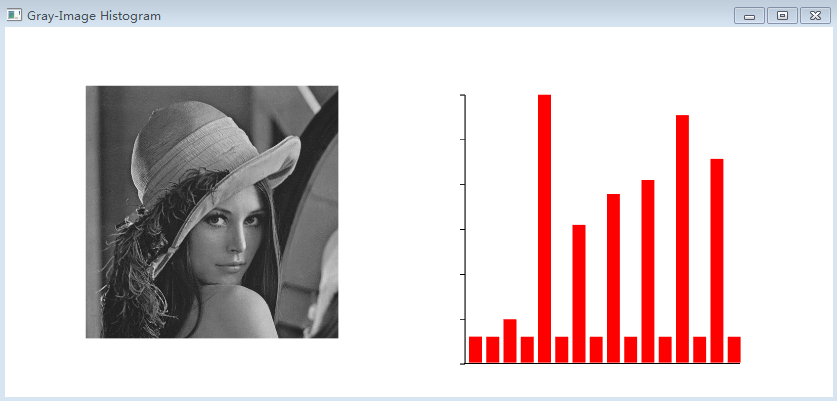2.参看资料

1.《C++ primer》 2.《The VTK User’s Guide – 11thEdition》 3.《The Visualization Toolkit – AnObject-Oriented Approach To 3D Graphics (4th Edition)》 4.  张晓东, 罗火灵. VTK图形图像开发进阶[M]. 机械工业出版社, 2015.


展开全文• 该算法中，按从左至右、从上至下的顺序，对整幅图像进行扫描，通过比较每个前景像素的邻域进行连通区标记，并创建等效标记列表。最后，合并等效标记列表，并再次扫描图像以更新标记。算法的优点的是通俗易懂，缺点是...c语言 算法
• 通过泡沫图像统计建模,实现了基于图像空间结构感知的浮选泡沫状态自动识别与客观评价。首先,采用Weibull分布建立了泡沫图像各方向边缘响应结构的统计分布模型,有效获取了泡沫图像空间结构的统计分布细节;然后,通过...
• 图像像素统计程序图像像素统计程序图像像素统计程序图像像素统计程序图像像素统计程序
• ## 图像的统计特征

万次阅读 2016-11-22 21:59:26相关系数
• ## 图像的统计方法

千次阅读 2018-01-06 10:01:58
前面我们提到，用均值滤波器、中值滤波器、高斯滤波器等可以降低图像中的噪声，其副作用是带来了图像模糊。我们降低噪声的目的，当然是为了提高图像的质量，这是“图像增强”的一个重要应用。 那图像质量怎么评价呢...opencv
• 计算图像基本统计指标--数字图像处理。通过对Matlab的讲解和实践，从而掌握Matlab基本语法和使用技巧，熟悉Matlab图像处理工具箱的使用方法；了解图像统计指标的犯法及其在图像处理中的意义。
• ## 图像的特征统计

千次阅读 2019-05-10 13:06:00
1.图像基本统计分析量 设f(i,j)f(i,j)f(i,j)表示大小为M×NM \times NM×N的数字图像，则该图像的基本统计量如下。 图像的信息量 一幅图像如果共有k种灰度值，并且各灰度值出现的概率分别为p1，p2，···，pk，根据...
• 用C语言实现raw格式图像的读取及对其灰度分布进行统计
• 血液红细胞识别与统计系统（ 血液红细胞识别与统计系统的实例，介绍图像处理、图像分析、图像识别。）
• 基于统计图像增强,基于统计图像增强MATLAB
• 这是一幅基因芯片的荧光图像，检测图像的ROI区域，对这个区域内的阴性点（弱）和阳性点（强）的数量进行统计，并标出点的位置。 ROI区域检测： 思路： (1)观察到图像对比度很低，首先对图像进行对比度增强 (2)图像...opencv
• 提出一种基于图像空间结构统计建模的复杂纹理图像模式识别方法. 从理论上分析了复杂纹理图像空间结构的韦伯分布过程, 通过构造多尺度全向高斯导数滤波器, 获得复杂纹理图像在不同观测尺度上的全方向空间结构统计建模...
• 前面我们提到，用均值滤波器、中值滤波器、高斯滤波器等可以降低图像中的噪声，其副作用是带来了图像模糊。我们降低噪声的目的，当然是为了提高图像的质量，这是“图像增强”的一个重要应用。 那图像质量怎么评价呢...
• 研究了重叠区域图像分割和参数统计的算法，提出综合利用阈值法和距离变换，并采用所设计的两个计算流程在对图像进行分割的同时完成参数统计。计算机模拟结果表明，整个算法不需要人工干预，易于软件和硬件实现。
•class python
• 该程序对SAR图像的背景杂波建立了多种统计模型，如对数正态分布、韦布尔分布、瑞利分布、gamma分布等，分别计算其拟合参数。并通过k-s准则，绝对值准则，峰度等选择最佳的分布模型和参数估计，并把各种分布图进行......